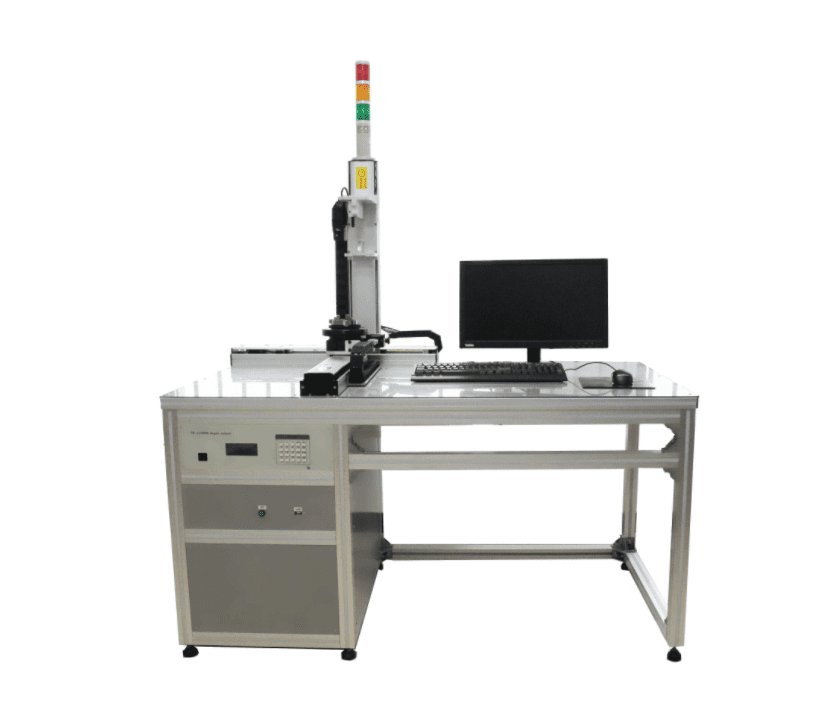#### products categoriesDownload file and products catalogue for equipment.

• Tel : 18030236818
• Fax : +86-592 5237901
• Email : dexing@china-dexing.com

# Constant field measurementThe measurement of a time - independent direct - current magnetic field. There are seven kinds of commonly used measuring instruments.

① Torque magnetometer: magnetometer for short. The force effect of a magnetic field is used to measure the strength of a magnetic field or the magnetization of a material.
② Fluxmeter and impulse galvanometer (see galvanometer) : used for impulse method (see soft magnetic material measurement) to measure the flux and flux density. When measuring, the magnetic flux in the detection coil must be changed artificially.

③ Rotating coil magnetometer: in the measured constant magnetic field, place a small detection coil, and make it rotate at a uniform speed. By measuring the electromotive force of the coil, the flux density or magnetic field strength can be calculated. The measurement range is from 0.1 mT to 10 mT. The error is 0.1 to 1%. The detection coil can also be turned suddenly or quickly moved to the field free area to measure the magnetic flux density according to the principle of impact method.
(4) fluxgate magnetometer: made of high permeability soft magnetic materials at the same time by alternating and constant magnetic field of the core, due to the non-linearity of the magnetization curve, and the core works in the asymmetric area of the curve, so that the voltage induced by the detection coil winding on the core contains even harmonic components, especially the second harmonic. This harmonic voltage is proportional to the strength of the constant magnetic field. The magnetic field strength is calculated by measuring the harmonic voltage of the detection coil. The principle structure of fluxgate magnetometer is shown in the figure. The two cores in the probe are made of a soft magnetic alloy with high permeability. AC excitation coils are wound on each core, and the detection coils are wound on the two cores. Two AC excitation coils are connected in series and powered by an oscillator. The magnetic field generated in the two cores is H ~, but in opposite directions. In this way, the fundamental and odd harmonic voltages induced in the detection coil cancel each other out. When for H0 measured probe in intensity constant magnetic field, respectively by two core H0 + H ~ and H0 - H - that is, alternating with constant magnetic field superposition effect, thus in detecting coil accidentally harmonic voltage, the frequency selective amplification and synchronous detection link, take the second harmonic voltage, and measured the values of the constant magnetic field intensity is proportional to the H0. The fluxgate magnetometer is highly sensitive with a resolution of 100 pT. It is mainly used to measure weak magnetic fields. It is widely used in geological, Marine and space technologies. The optical pump magnetometer developed in 1960s and 1970s and the superconducting quantum magnetometer made of superconducting quantum interference device (SQUids) are more sensitive

⑤ Hall effect magnetometer: the rectangular semiconductor wafer is placed in a magnetic field perpendicular to the wafer plane (magnetic flux density is B). If the DC current I is passed between the relative two end faces of the wafer, the electromotive force E(Hall effect) will be generated between the corresponding points on the other two ends of the wafer. When I is constant, E is proportional to B, and the proportional coefficient is related to the sheet width B, length l and thickness d, as well as the material used. This property of materials is also known as magnetic sensitivity. The Hall effect magnetometer can measure the flux density in the range of 1 microterre to 10 microterre. The error is 0.1 to 5%. Hall plates can be made thin and small, can be inserted into narrow gaps for measurement, and can also be used to measure non-uniform magnetic fields. In addition to Hall plate, there are bismuth helix, magnetic diode and so on.
6 nuclear magnetic resonance (NMR) magnetometer: the magnetic moment of an atomic nucleus, under the action of the magnetic flux density B will revolve around precession magnetic field direction, the precession frequency ƒ 0 = gamma B (gamma for gyromagnetic than that for certain substances, it is a constant), if in a small alternating magnetic field applied perpendicular to the direction of the B, when its frequency equals the ƒ 0, will produce resonance absorption phenomenon, namely, nuclear magnetic resonance (NMR). The magnetic flux density or magnetic field strength can be accurately calculated from the resonance frequency. The magnetometer has a measuring range of 0.1 millisit to 10 terre. Accuracy is very high, the error is less than 10-4 ~ 10-5, commonly used to provide a standard magnetic field and as a calibration standard.

⑦ magnetic potential meter: used to measure the magnetic potential difference between two points in space A and B, such as a uniform magnetic field, can be converted to the magnetic field strength. A potentiometer can also be used to measure the strength of the magnetic field inside a material. Since the tangent component of magnetic field strength at the interface of magnetic materials is equal, the magnetic field strength measured by a potentiometer along the space of the material surface is the tangent component of magnetic field strength inside the material. The structure of the magnetic potentiometer is the fine insulated wire evenly wound on the non-magnetic soft tape or hard piece, the former is called soft magnetic potentiometer; The latter is called hard magnetic potentiometer. The measuring instrument adopts impulse galvanometer or flux meter. For a constant magnetic field, the flux linked by the potentiometer must be changed during the measurement. If the measured magnetic field is uniform, the magnetic field intensity can be converted from the magnetic potential difference. The potentiometer can be calibrated in a standard uniform magnetic field and graduated according to the magnetic field strength value.